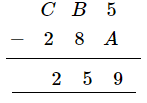# Replace A, B, C by suitable numerals.

Question:

Replace A, B, C by suitable numerals.Solution:

$5-A=9$

This implies that 1 is borrowed.

We know:

$15-6=9$

$\therefore A=6$

$B-5=8$

This implies that 1 is borrowed.

$13-5=8$

But 1 has also been lent

$\therefore B=4$

$C-2=2$

This implies that 1 has been lent.

$\therefore C=5$

$\therefore A=6, B=4$ and $C=5$